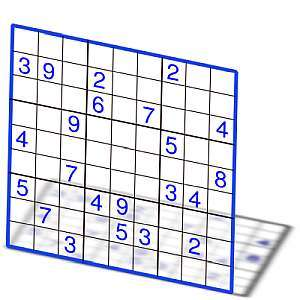# Ceil

Instructions: Use this ceil calculator to compute any the ceil of any numeric expression you provide, showing all the steps. Please type in a numeric expression for which you want to compute the ceil in the form box below.Enter the numeric expression you want to compute the ceil for (Ex: 2/3, etc.)

## Ceil calculator

This ceil calculator will allow you to compute the ceiling of any numeric expression you provide, showing all the steps. All you need to provide a valid numeric expression, which could be a decimal like 3.46, or you can provide a fraction like 13/4, or ultimately you can provide any valid numeric expression like 1/3 + 1/4.

Once a valid expression has been provided in the form box, you can click on "Calculate" button, and you will be provided with the ceil calculation, with all the steps shown.

Ceil calculation is usually a very simple operation, especially if a decimal number is provided. If a more complex expression is provided, you will need get a numeric value before computing its ceiling.## What is the ceil of a number

The ceil or ceiling of a number is the smallest integer that is greater than or equal to the given number. In simple words, the ceil of a number is the "next" integer that is 'above' the number. When we say 'above' we mean 'above or equal'

With this definition, the ceil of an integer number is the number itself. For example, the ceil of 3 is 3. When the number is not an integer, we directly look for the next integer above the number. For example, the ceil of 4.3 is 5.

## What are the steps for finding the ceil?

• Step 1: Identify the number you want to compute the ceil, and call it x
• Step 2: If x is integer, then the ceil is also x, and the calculation is finished
• Step 3: If x is not an integer, the ceil is the 'next' integer: Compute the value of x + 1, and drop all of its decimals, and you have arrived to the ceil of x

For example, consider x = 4.1. Is x = 4.1 integer? No, so then we compute x + 1 = 5.1, and we drop the decimals of 5.1, so we get 5. Hence, the ceil of x = 4.1 is 5.

Notice that the ceil is not the same as rounding a number. Indeed the ceil of 4.1 is 5, but if you rounded 4.1, you get 4.

## How to use this ceil calculator?

All you need to do is to type of the number or expression you want to compute the ceil of. Our ceil calculator will follow the steps described above to compute the ceil.

First, the calculator will assess whether the number provided is integer, and if it is not, then it proceeds as described by step 3.

## What are applications for ceil?

It is common to find ceil on applications. Often times it happens that we are looking for integer variables that are not smaller than a certain value that is not necessarily integer, in which case, the idea of ceil appears naturally.

For example, this can often happens in optimization problems. Usually those problems are solved in Excel, which conveniently has a =CEIL() function included.### Example: Ceil example

Calculate the ceil of: $$\frac{1}{3} + \frac{7}{4} - \frac{5}{6}$$

Solution: We need to calculate the ceiling of the following expression: $$\displaystyle \frac{1}{3}+\frac{7}{4}-\frac{5}{6}$$.

The expression can be further reduced, the following are the simplification steps:

$$\displaystyle \frac{1}{3}+\frac{7}{4}-\frac{5}{6}$$
$$=$$
$$\displaystyle \frac{5}{4}$$
Grouping and operating all the integer terms and fractions: $$\displaystyle \frac{ 1}{ 3}+\frac{ 7}{ 4}-\frac{ 5}{ 6}=\frac{ 1}{ 3} \times \frac{ 4}{ 4}+\frac{ 7}{ 4} \times \frac{ 3}{ 3}-\frac{ 5}{ 6} \times \frac{ 2}{ 2}=\frac{ 4+7 \times 3-5 \times 2}{ 12}=\frac{ 4+21-10}{ 12}=\frac{ 15}{ 12}=\frac{ 3 \times 5}{ 3 \times 4}=\frac{ \cancel{ 3} \times 5}{ \cancel{ 3} \times 4}=\frac{ 5}{ 4}$$

Notice that $$\displaystyle \frac{5}{4} = 1.25$$, so then its integer part is $$1$$, so then adding 1 to the integer part, we get that the ceil of $$\displaystyle \frac{5}{4}$$ is $$2$$.

Conclusion: The ceil of the provided expression $$\displaystyle \frac{1}{3}+\frac{7}{4}-\frac{5}{6}$$ is equal to $$2$$.

### Example: Ceil calculator

Calculate the ceil of 3.0000001

Solution: In this case we have the expression: $$\displaystyle 3.0000001$$.

Notice that the number has decimals, and its integer part is $$3$$, so then adding 1 to the integer part, we get that the ceil of $$\displaystyle 3$$ is $$4$$.

Conclusion: The ceil of $$\displaystyle 3.0000001$$ is equal to $$4$$.

### Example: Another fraction calculation

Can a number have the same ceil and floor?

Solution: Yes, indeed, and that only happens for an integer number.

## More algebra calculators

Tightly related to the idea of ceil, you can use this floor calculator and this round calculator.

Also, you can use this expression evaluator to get the numerical resulting number associated to it. For other specific type of expressions, you may be interested in this fraction calculator and root calculator.